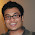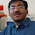### Shortest Curve dividing Equilateral Triangle

Source: Asked to me by Dinesh Krithivasan (IITM Alumnus, Phd University of Michigan, Senior Qualcomm Engineer)

Finally a geometry problem for the blog. :) Thanks Dinesh! :)

Problem:
We have an equilateral triangle ABC of unit side length. We want to find a curve C of the smallest length that cuts this triangle into 2 halves of equal area. Obviously, the altitude of length sqrt(3)/2 will do the job but can we do better? Note that there is no other restriction on C - it need not pass through any of the triangle vertices for instance.

1.Line parallel to a base of length 1/sqrt(2) is an improvement over the altitude. Though, I still need to prove that it is the shortest length.

2.suppose that the curve starts from AB and ends on AC.

appropriately adjoin six identical copies of triangle ABC in a circlular way with position of A fixed at center so that we get a closed curve enclosing a fixed area. for a given area, perimeter of a curve is known to be least for circle.

So, curve should be a circular arc with center at A and radius of appropriate length.

3.Parallel to the base at a height 1/sqrt(2) of the altitude from the vertex. Length of this curve (line) would be 1/sqrt(2) ~ 0.707 < sqrt(3)/2 = 0.866 in the example you illustrated. Or can we do better?

4.Parallel to the base forming a similar triangle of area half of the original. Therefore length of this curve (line) would simply by 1/sqrt(2) ~ 0.707.

5.One way in which we can do better(than altitude) is to draw a line parallel to the base that divides the triangle into two equal areas. In this case, the length of the dividing line would be 1/sqrt(2). However I am not sure if this is the best we can do.

6.correction. Just a circular arc from the top vertex. Set pi*(r^2)/6 = sqrt(3)/8. Solve for r. And get 2pi*r/6. Works out to sqrt(3*pi*sqrt(3))/6 = 0.673.

7.On second thought, may be we can draw an arc from any one vertex that divides the triangle into two equal areas. This would be better than the previous approach, as circle is the most efficient curve (in terms of area packed for same perimeter).

8.i had assumed that one of the two halves will contain a single vertex. this need not be true.

if the curve starts and ends on BC, then, all three vertices will be in one half.

for this case, we can reflect the triangle about BC to get a closed curve with a fixed area. for minimum perimeter, this must be a circle. but the length of semi-circular arc in this case will be greater.

9.Ok..here goes...

Triangle ABC is equilateral.
Assume the centre of the arc to be at infinity...
length 'L1' of arc (a straight line parallel to BC)..centre is on line perpendicular to BC.

L1 = 1/sqrt(2)

Imagine centre to be at A, radius R

length of arc, L2.

pi*R*R/6 = root(3)/8
R = 0.6430

going on..imagine the centre to be on AB...at a infinitesimally small distance from A.
length of arc = L3
arc intersecting AC at D and AB at E.

Area = [ADO] + arc[ODE].. O is the centre

imagine the radius to be 'r' .

now [referencing to R from before]

pi*R*R/6 = pi*r*r/6 + r*r*q

sq(L2/L3) = sq[(pi/6)R / {(pi/6)+q}r]
eventually

sq(L2/L3) = 1 / [1+ 6q/pi] ....
unless we did a calculation mistake.

implying....moving a delta distance off the altitude..the length of the arc increase..
[though the points on the altitude might be a local minima...so that moving delta distance increases the arc]

Bringing over centre closer to BC..

imagine O such that, the circle passes through A.

L4 = 0.5845

Let's try to move closer.
O` lies on the altitude...closer to BC that O.
And try it for yourself...left as homework

10.Wow.. Chera was spot on, although for completeness he could have added a third case of the circle in the triangle.

http://mathproblems123.files.wordpress.com/2011/11/lalescu.pdf

11.I am here to discuss about triangles as-Similar triangles both have equal angles but sides have different sizes. Similar triangles are also known as equiangular triangle.
What is the Area of a Triangle